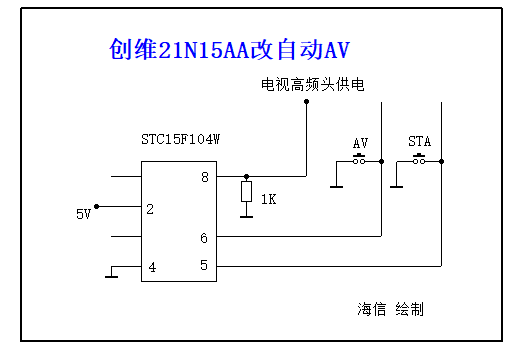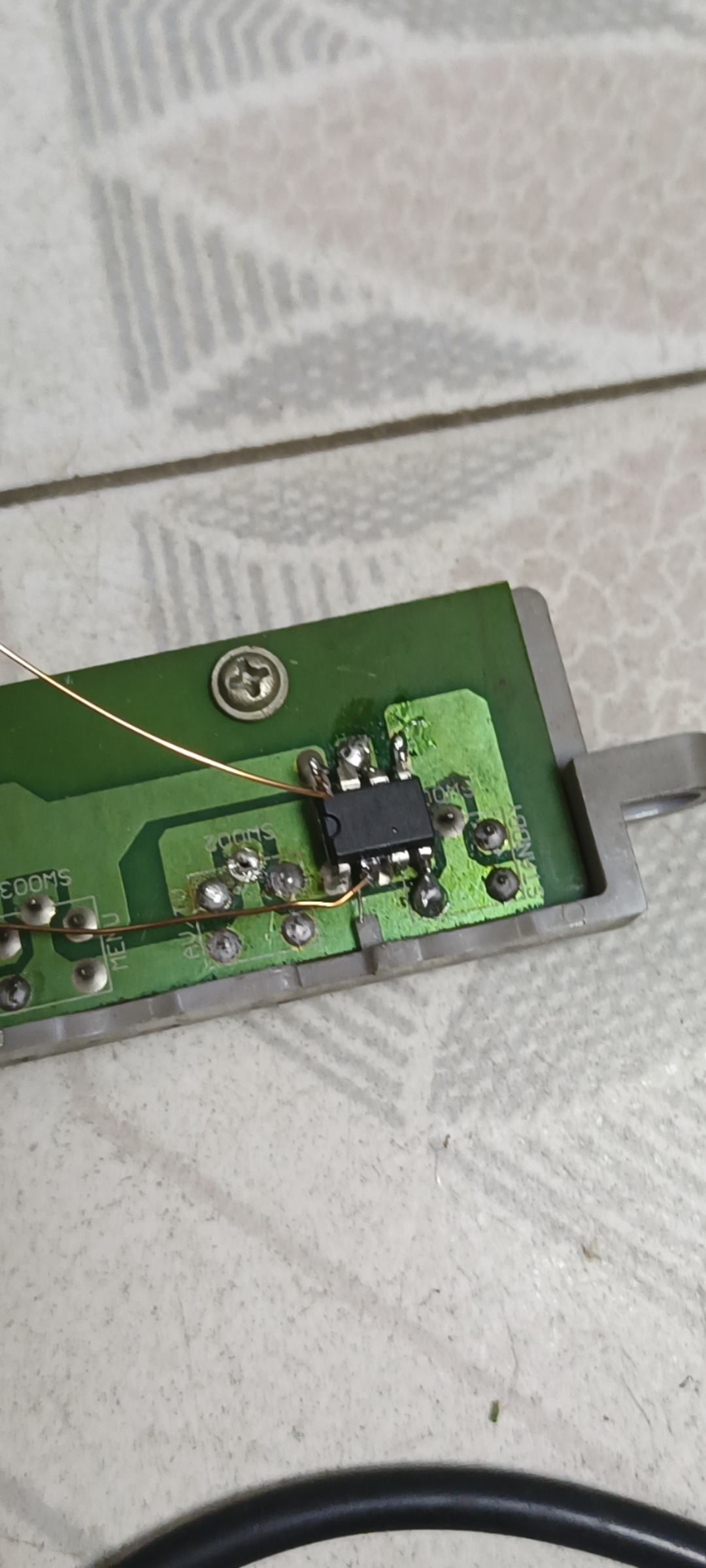```#include <STC15.H> //头文件

sbit ON_key = P3^0;	 //5脚，做二次开机键
sbit AV_key = P3^1;	 //6脚 做AV键
sbit TEST = P3^3;	//8脚，高低电平检测

void Delayms(unsigned int ms) //XmS@12.000MHz//X毫秒延时
{
unsigned char i, j;
while(ms--)
{i = 12;
j = 169;
do{
while (--j);
}
while (--i);
}
}

//****主程序

void main(void)
{
P3M1=0X07; //0000 0111  //P3.0,P3.1,P3.2为开漏，其余为标准弱上拉
P3M0=0X07; //0000 0111
AV_key=ON_key=TEST=1;

Delayms(10000);   //延迟10秒让机器CPU等都完成
if(TEST==0) //8脚检测判断,这里没开机的话就模拟按键开机
{
ON_key= 0; //P3^0,5脚。
Delayms(200);
ON_key= 1;
Delayms(10000);
}
AV_key= 0; //P3^1,6脚。
Delayms(200);
AV_key= 1;    //模拟按键按AV
while(1){}    //死循环维持状态
}```• 友情链接